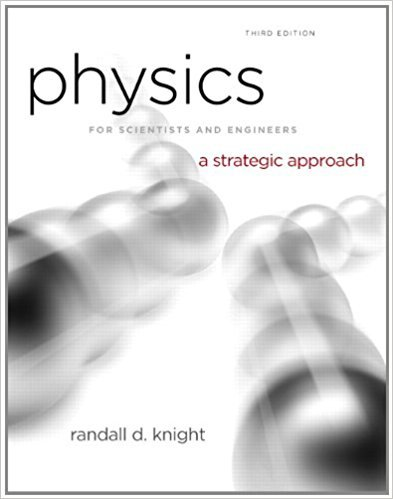×
Get Full Access to Physics For Scientists And Engineers: A Strategic Approach With Modern Physics - 3 Edition - Chapter 15 - Problem 14e
Get Full Access to Physics For Scientists And Engineers: A Strategic Approach With Modern Physics - 3 Edition - Chapter 15 - Problem 14e

×

# Figure P15.18 is a snapshot graph of a wave at t = 0 s.ISBN: 9780321740908 69

## Solution for problem 14E Chapter 15

Physics for Scientists and Engineers: A Strategic Approach with Modern Physics | 3rd Edition

• Textbook Solutions
• 2901 Step-by-step solutions solved by professors and subject experts
• Get 24/7 help from StudySoup virtual teaching assistantsPhysics for Scientists and Engineers: A Strategic Approach with Modern Physics | 3rd Edition

4 5 1 268 Reviews
18
1
Problem 14E

Problem 14E

Figure P15.18 is a snapshot graph of a wave at t = 0 s. What are the amplitude, wavelength, and frequency of this wave?Step-by-Step Solution:

Solution 14 E

Step 1 of 2

We have to find the amplitude, wavelength, and frequency of a wave given in the figure.The amplitude of the wave is equal to the vertical distance of the peak from theaxis in the given figure.The wavelength is equal to the horizontal distance between the successive peaks of the wave.Step 2 of 2

##### ISBN: 9780321740908

Unlock Textbook Solution